# 前言

• 自定义View是Android开发者必须了解的基础；而Path类的使用在自定义View绘制中发挥着非常重要的作用
• 网上有大量关于自定义View中Path类的文章，但存在一些问题：内容不全、思路不清晰、简单问题复杂化等等
• 今天，我将全面总结自定义View中Path类的使用，我能保证这是市面上的最全面、最清晰、最易懂的
1. 文章较长，建议收藏等充足时间再进行阅读
2. 阅读本文前请先阅读自定义View基础 - 最易懂的自定义View原理系列

# 目录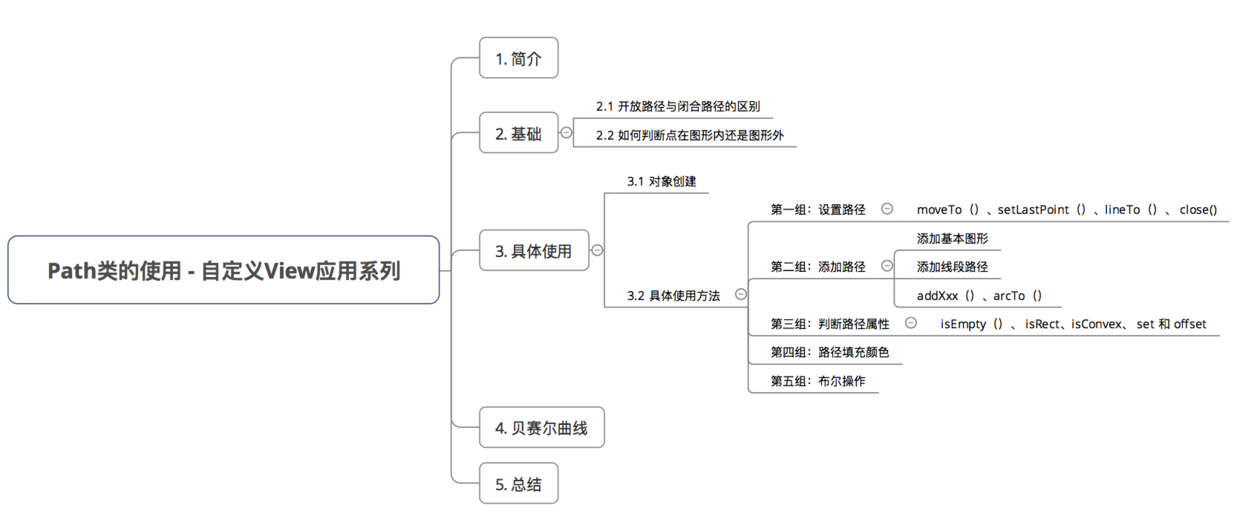#1. 简介

• 定义：路径，即无数个点连起来的线
• 作用：设置绘制的顺序 & 区域

Path只用于描述顺序 & 区域，单使用Path无法产生效果

• 应用场景：绘制复杂图形（如心形、五角星等等）

Path类封装了由直线和曲线（2、3次贝塞尔曲线）构成的几何路径。

# 2. 基础

### 2.1 开放路径与闭合路径的区别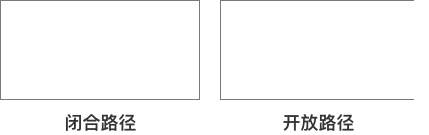### 2.2 如何判断点在图形内还是图形外

• 判断方法分为奇偶规则 & 非零环绕规则，具体介绍如下：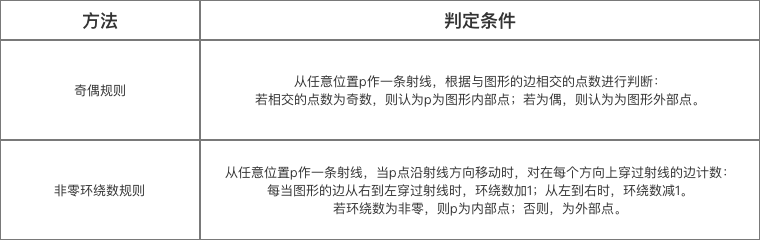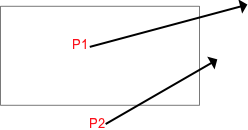• p1发出的射线与图形相交1个点，即奇数点，所以P1点在图形内
• p2发出的射线与图形相交2个点，即偶数点，所以P2点在图形内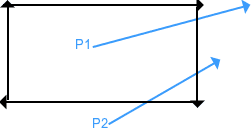• p1发出的射线与图形相交1个点，矩形的右侧线从左边射到右边，环绕数－1，最终环绕数为－1，故p1在图形内部。
• p2发出的射线与图形相交2个点：矩形的右侧边从左边射到右边
环绕数－1；矩形的下侧边从右边射到左边，环绕数+1，最终环绕数为0.故p2在图形外部

# 3. 具体使用

## 3.1 对象创建

    // 使用Path首先要new一个Path对象
// Path的起点默认为坐标为(0,0)
Path path = new Path();
// 特别注意：建全局Path对象，在onDraw()按需修改；尽量不要在onDraw()方法里new对象
// 原因：若View频繁刷新，就会频繁创建对象，拖慢刷新速度。



## 3.2 具体方法使用

### 第一组：设置路径


// 设置当前点位置
// 后面的路径会从该点开始画
moveTo(float x, float y) ；

// 当前点（上次操作结束的点）会连接该点
// 如果没有进行过操作则默认点为坐标原点。
lineTo(float x, float y)  ；

// 闭合路径，即将当前点和起点连在一起
// 注：如果连接了最后一个点和第一个点仍然无法形成封闭图形，则close什么也不做
close() ；


• 可使用setLastPoint（）设置当前点位置（代替moveTo（）
• 二者区别：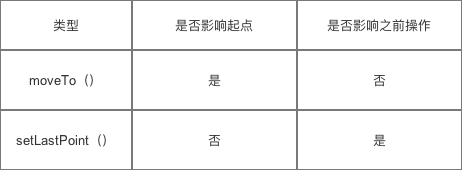// 使用moveTo（）
// 起点默认是(0,0)
//连接点(400,500)
path.lineTo(400, 500);

// 将当前点移动到(300, 300)
path.moveTo(300, 300) ;

//连接点(900, 800)
path.lineTo(900, 800);

// 闭合路径，即连接当前点和起点
// 即连接(200,700)与起点2(300, 300)
// 注:此时起点已经进行变换
path.close();

// 画出路径
canvas.drawPath(path, mPaint1);

// 使用setLastPoint（）
// 起点默认是(0,0)
//连接点(400,500)
path.lineTo(400, 500);

// 将当前点移动到(300, 300)
// 会影响之前的操作
// 但不将此设置为新起点
path.setLastPoint(300, 300) ;

//连接点(900,800)
path.lineTo(900, 800);

//连接点(200,700)
path.lineTo(200, 700);

// 闭合路径，即连接当前点和起点
// 即连接(200,700)与起点(0，0)
// 注:起点一直没变化
path.close();

// 画出路径
canvas.drawPath(path, mPaint1);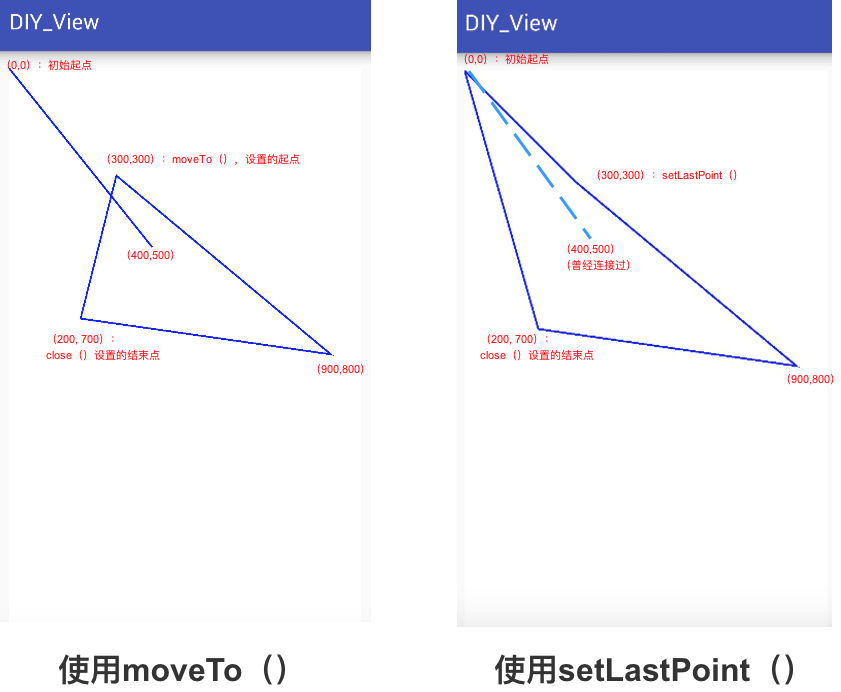### 关于重置路径

• 重置Path有两个方法：reset（）rewind（）
• 两者区别在于：

Path.reset（）
Path.rewind（）
1. FillType影响显示效果；数据结构影响重建速度
2. 所以一般选择Path.reset（）

# 第二组: 添加路径

### 2.1 添加基本图形

• 作用：在Path路径中添加基本图形

• 具体使用

// 添加圆弧
// 方法1
public void addArc (RectF oval, float startAngle, float sweepAngle)

//  startAngle：确定角度的起始位置
//  sweepAngle ： 确定扫过的角度

// 方法2
// 与上面方法唯一不同的是：如果圆弧的起点和上次最后一个坐标点不相同，就连接两个点
public void arcTo (RectF oval, float startAngle, float sweepAngle)

// 方法3
// 参数forceMoveTo：是否将之前路径的结束点设置为圆弧起点
// false：在新的起点画圆弧，但会连接之前路径的结束点与圆弧起点，即与之前路径有交集（同arcTo（3参数））
public void arcTo (RectF oval, float startAngle, float sweepAngle, boolean forceMoveTo)
// 下面会详细说明

// 加入圆形路径
// 起点：x轴正方向的0度
// 其中参数dir：指定绘制时是顺时针还是逆时针:CW为顺时针，  CCW为逆时针
// 路径起点变为圆在X轴正方向最大的点

// 加入椭圆形路径
// 其中，参数oval作为椭圆的外切矩形区域

// 加入矩形路径
// 路径起点变为矩形的左上角顶点

//加入圆角矩形路径

addRoundRect(RectF rect, float rx, float ry, Path.Direction dir)

//  注：添加图形路径后会改变路径的起点


dir = Direction = 图形的方向，为枚举类型：

• CW：clockwise，顺时针
• CCW：counter-clockwise，逆时针

• 添加图形时确定闭合顺序(各个点的记录顺序)
• 图形的渲染结果(是判断图形渲染的重要条件)

**图形绘制的本质：先画点，再将点连接起来。**所以，点与点之间是存在一个先后顺序的；顺时针和逆时针用于确定这些点的顺序。

  // 为了方便观察,平移坐标系
canvas.translate(350, 500);
// 顺时针

// 逆时针
canvas.drawPath(path,mPaint1);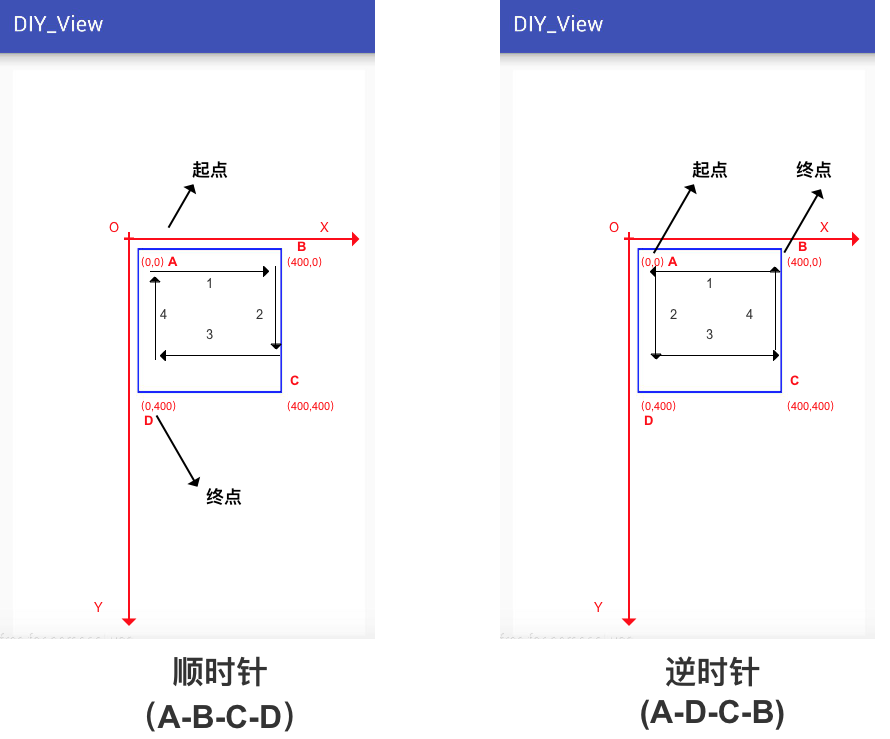// 轨迹1
// 将Canvas坐标系移到屏幕正中
canvas.translate(400,500);

// 起点是(0,0)，连接点(-100,0)
path.lineTo(-100,0);
// 连接点(-100,200)
path.lineTo(-100,200);
// 连接点(200,200)
path.lineTo(200,200);
// 闭合路径，即连接当前点和起点
// 即连接(200,200)与起点是(0,0)
path.close();

// 画出路径
canvas.drawPath(path,paint);
// 具体请看下图

// 轨迹2
// 将Canvas坐标系移到屏幕正中
canvas.translate(400,500);

// 起点是(0,0)，连接点(-100,0)
path.lineTo(-100,0);
// 画圆：圆心=(0,0)，半径=100px
// 此时路径起点改变 = (0,100)（记为起点2）
// 起点改变原则：新画图形在x轴正方向的最后一个坐标
// 后面路径的变化以这个点继续下去

// 起点为：(0,100)，连接 (-100,200)
path.lineTo(-100,200);
// 连接 (200,200)
path.lineTo(200,200);

// 闭合路径，即连接当前点和起点（注：闭合的是起点2）
// 即连接(200,200)与起点2(0,100)
path.close();

// 画出路径
canvas.drawPath(path,paint);

// // 具体请看下图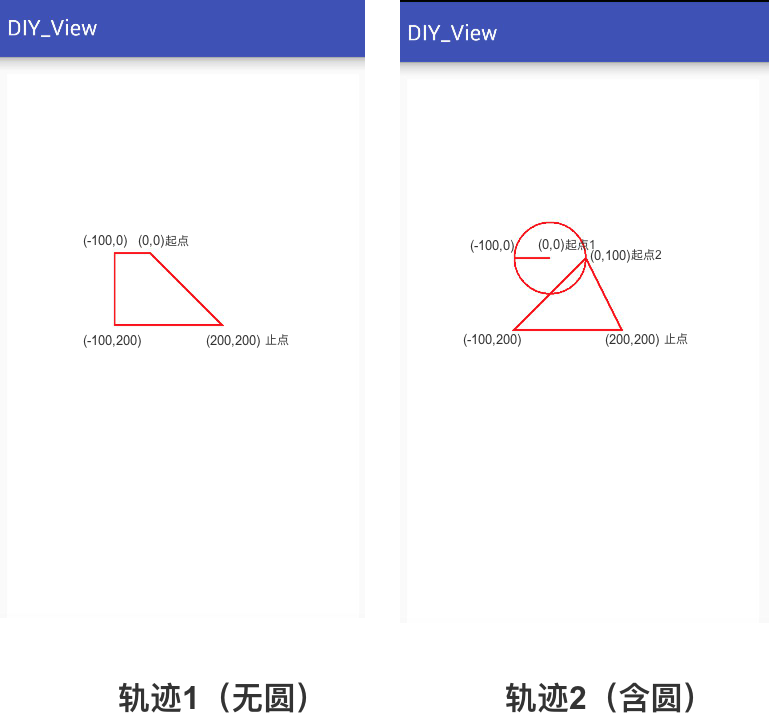// addArc
// 直接添加一个圆弧到path中
//  startAngle：确定角度的起始位置
//  sweepAngle ： 确定扫过的角度
public void addArc (RectF oval, float startAngle, float sweepAngle)

// arcTo
// 方法1
// 同样是添加一个圆弧到path
// 与上面方法唯一不同的是：如果圆弧的起点和上次最后一个坐标点不相同，就连接两个点
public void arcTo (RectF oval, float startAngle, float sweepAngle)

// 方法2
// 参数forceMoveTo：是否将之前路径的结束点设置为圆弧起点
// false：在新的起点画圆弧，但会连接之前路径的结束点与圆弧起点，即与之前路径有交集（同arcTo（3参数））
public void arcTo (RectF oval, float startAngle, float sweepAngle, boolean forceMoveTo)



// 将一个圆弧路径添加到一条直线路径里

// 为了方便观察,平移坐标系
canvas.translate(350, 500);

// 先将原点(0,0)连接点(100,100)
path.lineTo(50, 200);

// 添加圆弧路径(2分之1圆弧)

// 不连接最后一个点与圆弧起点
path.addArc(new RectF(200, 200, 300, 300), 0, 180);
// path.arcTo(oval,0,270,true);             // 与上面一句作用等价

// 连接之前路径的结束点与圆弧起点
path.arcTo(new RectF(200, 200, 300, 300), 0, 180);
// path.arcTo(oval,0,270,false);             // 与上面一句作用等价

// 画出路径
canvas.drawPath(path, mPaint1);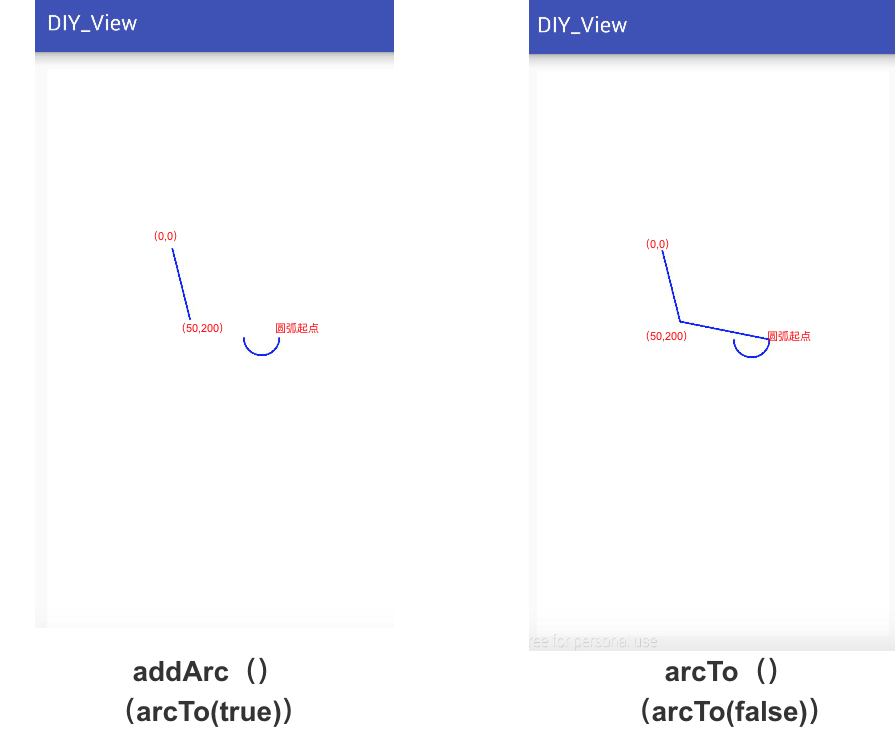## 2.2 添加路径

• 作用：合并路径

• 具体使用
    // 方法1

// 方法2
// 先将src进行（x,y）位移之后再添加到当前path
public void addPath (Path src, float dx, float dy)

// 方法3
// 先将src进行Matrix变换再添加到当前path
public void addPath (Path src, Matrix matrix)

// 实例：合并矩形路径和圆形路径

// 为了方便观察,平移坐标系
canvas.translate(350, 500);
// 创建路径的对象
Path pathRect = new Path();
Path  pathCircle = new Path();
// 画一个矩形路径
// 画一个圆形路径

// 将圆形路径移动(0,200),再添加到矩形路径里

// 绘制合并后的路径
canvas.drawPath(pathRect,mPaint1);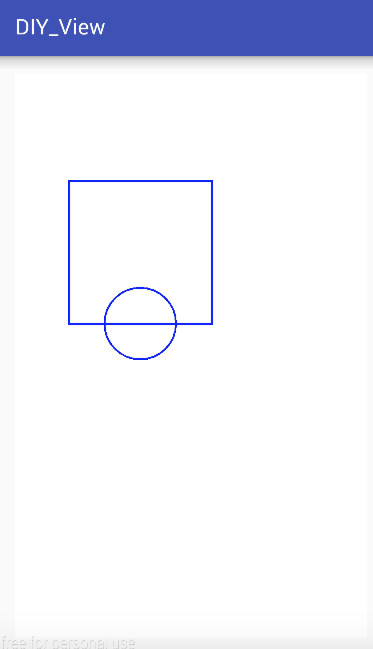### 第三组：判断路径属性

• 采用isEmpty（）、 isRect（）、isConvex（）、 set（） 和 offset（）组合

• 具体使用：

// 判断path中是否包含内容
public boolean isEmpty ()
// 例子：
Path path = new Path();
path.isEmpty();  //返回false

path.lineTo(100,100); // 返回true

// 判断path是否是一个矩形
// 如果是一个矩形的话，会将矩形的信息存放进参数rect中。
public boolean isRect (RectF rect)

// 实例
path.lineTo(0,400);
path.lineTo(400,400);
path.lineTo(400,0);
path.lineTo(0,0);

RectF rect = new RectF();
boolean b = path.isRect(rect);  // b返回ture,
// rect存放矩形参数，具体如下：
// rect.left = 0
// rect.top = 0
// rect.right = 400
// rect.bottom = 400

// 将新的路径替代现有路径
public void set (Path src)

// 实例
// 设置一矩形路径
Path path = new Path();

// 设置一圆形路径
Path src = new Path();

// 将圆形路径代替矩形路径
path.set(src);

// 绘制图形
canvas.drawPath(path,mPaint);

// 平移路径
// 与Canvas.translate （）平移画布类似

// 方法1
// 参数x,y：平移位置
public void offset (float dx, float dy)

// 方法2
// 参数dst：存储平移后的路径状态，但不影响当前path
// 可通过dst参数绘制存储的路径
public void offset (float dx, float dy, Path dst)

// 为了方便观察,平移坐标系
canvas.translate(350, 500);

// path中添加一个圆形(圆心在坐标原点)
path = new Path();

// 平移路径并存储平移后的状态
Path dst = new Path();
path.offset(400, 0, dst);                     // 平移

canvas.drawPath(path, mPaint1);               // 绘制path

// 通过dst绘制平移后的图形(红色)
mPaint1.setColor(Color.RED);
canvas.drawPath(dst,mPaint1);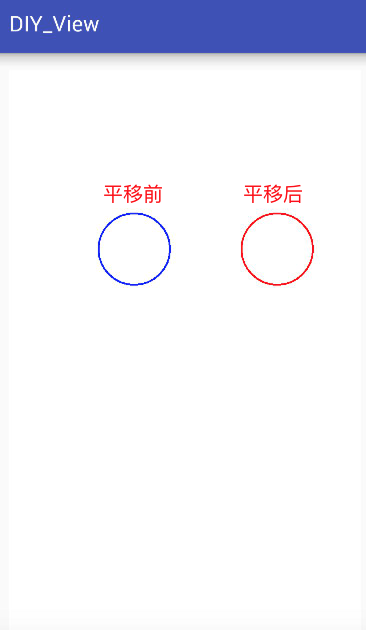### 第四组：设置路径填充颜色

• 在Android中，有四种填充模式，具体如下

EVEN_ODD 奇偶规则
INVERSE_EVEN_ODD 反奇偶规则
WINDING 非零环绕数规则
INVERSE_WINDING 反非零环绕数规则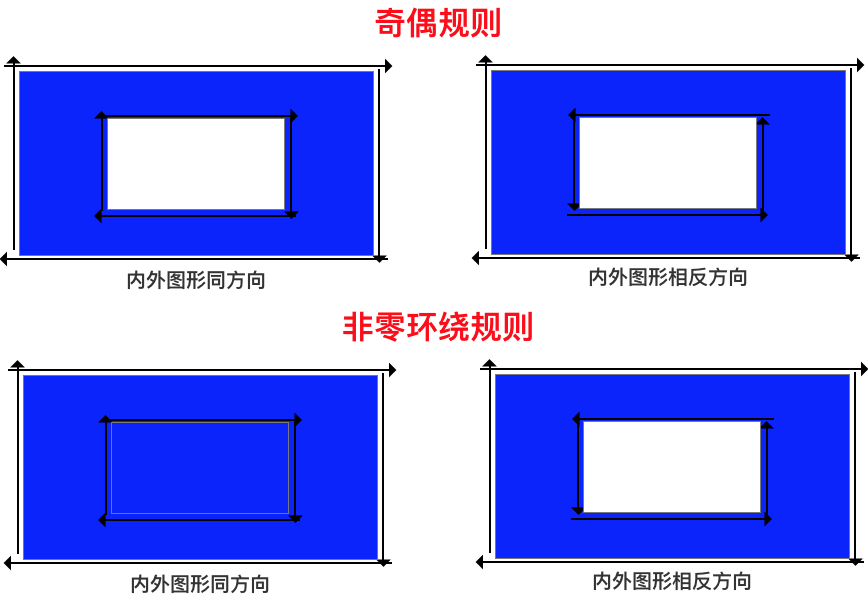• 具体使用
// 设置填充规则
path.setFillType（）
// 可填规则
// 1. EVEN_ODD：奇偶规则
// 2. INVERSE_EVEN_ODD：反奇偶规则
// 3. WINDING ：非零环绕数规则
// 4. INVERSE_WINDING：反非零环绕数规则

// 理解奇偶规则和反奇偶规则：填充效果相反
// 举例：对于一个矩形而言，使用奇偶规则会填充矩形内部，而使用反奇偶规则会填充矩形外部（下面会举例说明）

// 获取当前填充规则
path.getFillType（）

// 判断是否是反向(INVERSE)规则
path.isInverseFillType（）

// 切换填充规则(即原有规则与反向规则之间相互切换)
path.toggleInverseFillType（）




// 为了方便观察,平移坐标系
canvas.translate(350, 500);

// 在Path中添加一个矩形

// 设置Path填充模式为 奇偶规则
path.setFillType(Path.FillType.EVEN_ODD);

// 反奇偶规则
// path.setFillType(Path.FillType.INVERSE_EVEN_ODD);

// 画出路径
canvas.drawPath(path, mPaint1);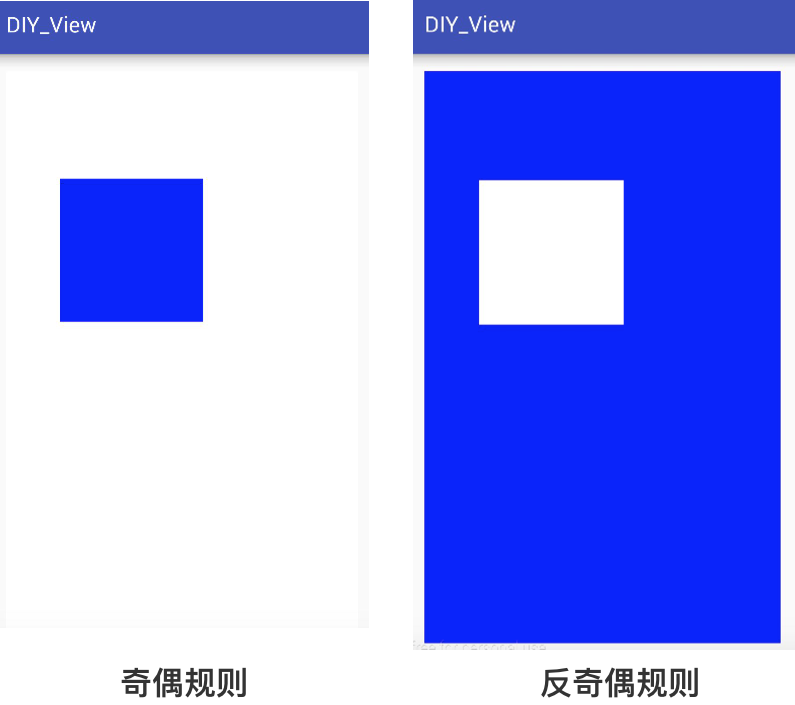// 为了方便观察,平移坐标系
canvas.translate(550, 550);
// 在路径中添加大正方形
// 逆时针

// 在路径中添加小正方形
// 顺时针
//        path.addRect(-200, -200, 200, 200, Path.Direction.CW);
//          设置为逆时针

// 设置Path填充模式为非零环绕规则
path.setFillType(Path.FillType.WINDING);
// 设置反非零环绕数规则
// path.setFillType(Path.FillType.INVERSE_WINDING);

// 绘制Path
canvas.drawPath(path, mPaint1);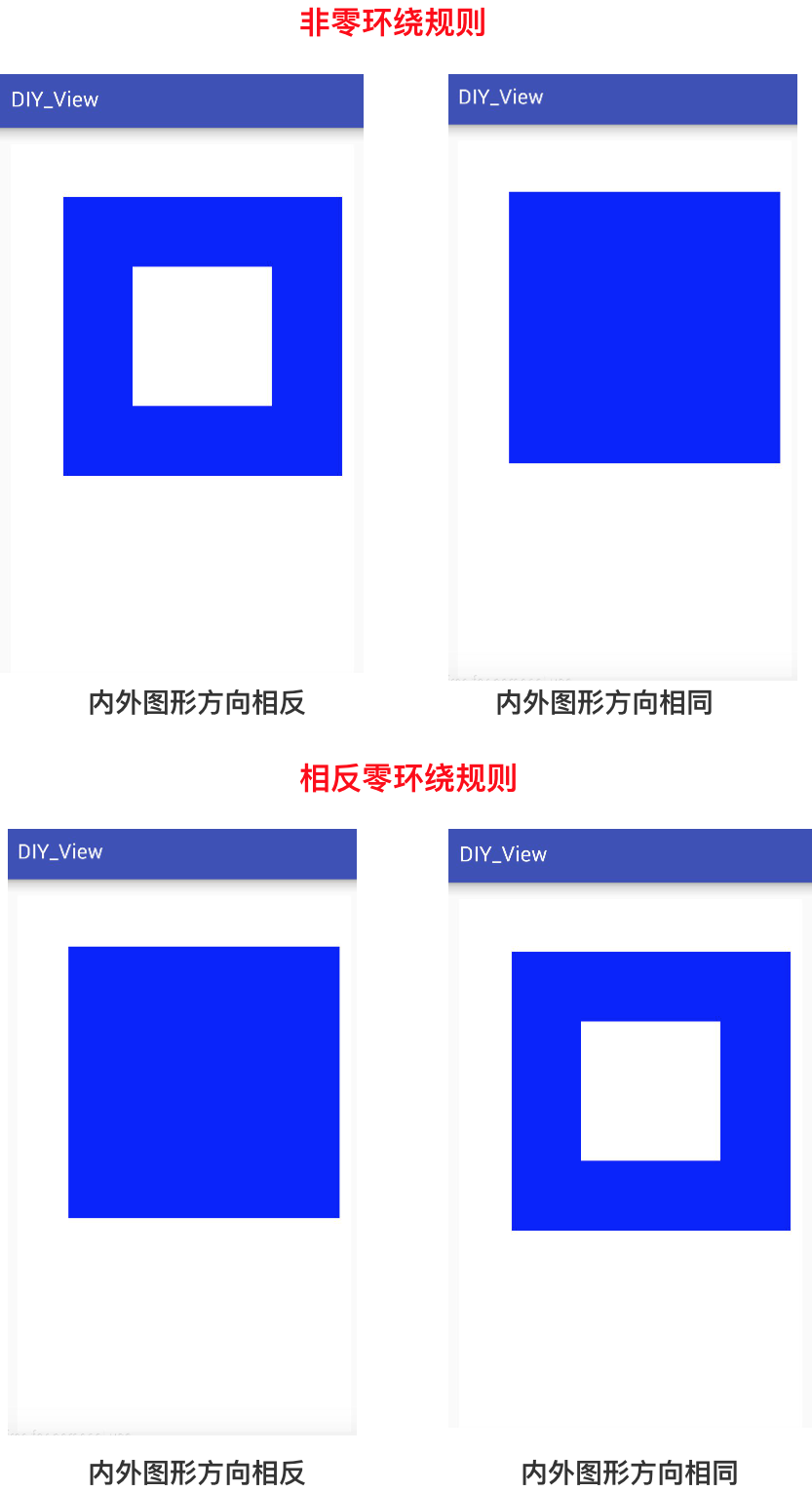# 第五组：布尔操作

• 作用：两个路径Path之间的运算
• 应用场景：用简单的图形通过特定规则合成相对复杂的图形。
• 具体使用
// 方法1
boolean op (Path path, Path.Op op)
// 举例
// 对 path1 和 path2 执行布尔运算，运算方式由第二个参数指定
// 运算结果存入到path1中。
path1.op(path2, Path.Op.DIFFERENCE);

// 方法2
boolean op (Path path1, Path path2, Path.Op op)
// 举例
// 对 path1 和 path2 执行布尔运算，运算方式由第三个参数指定
// 运算结果存入到path3中。
path3.op(path1, path2, Path.Op.DIFFERENCE)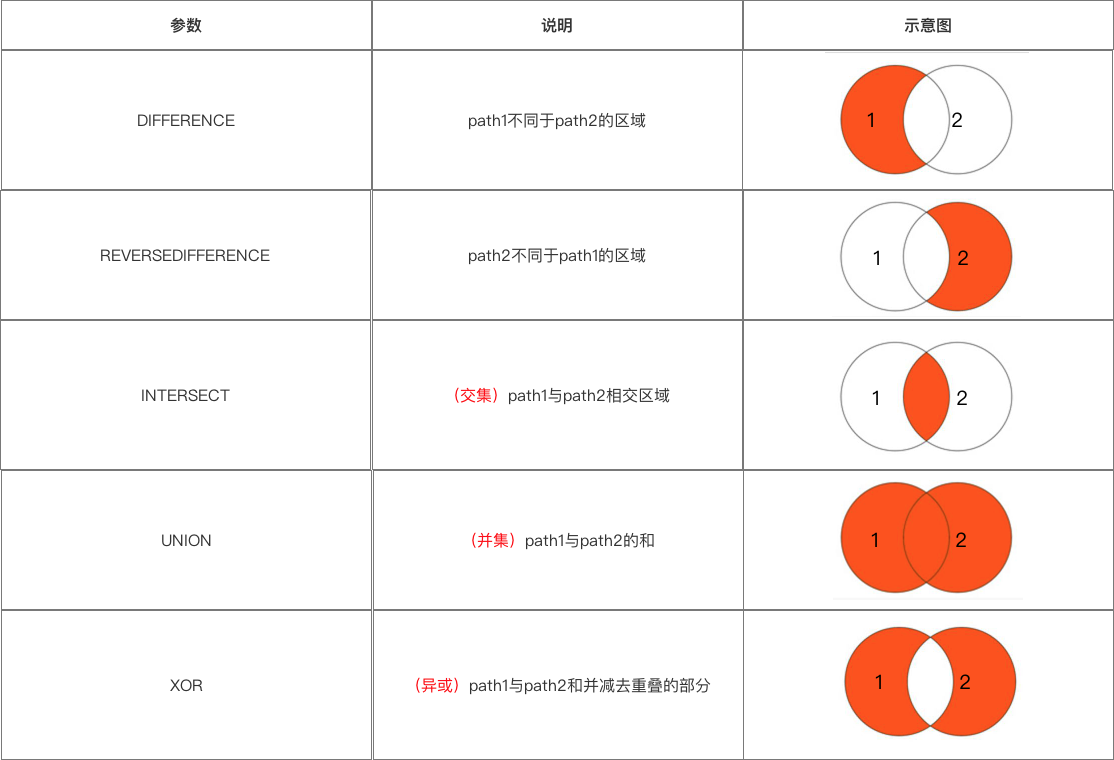// 为了方便观察,平移坐标系
canvas.translate(550, 550);

// 画两个圆
// 圆1:圆心 = (0,0),半径 = 100
// 圆2:圆心 = (50,0),半径 = 100

// 取两个路径的异或集
path1.op(path2, Path.Op.XOR);
// 画出路径
canvas.drawPath(path1, mPaint1);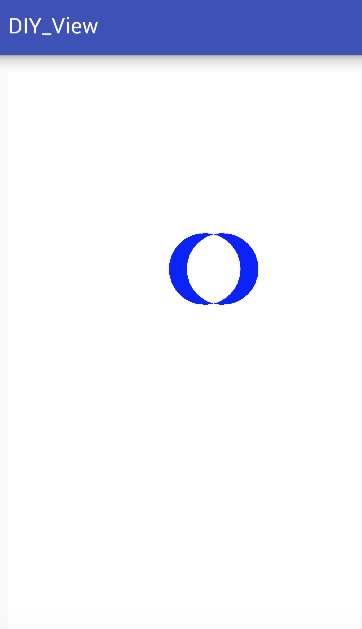# 4. 贝赛尔曲线

• 定义：计算曲线的数学公式
• 作用：计算并表示曲线

• 具体使用：贝塞尔曲线可通过1数据点和若干个控制点描述
1. 数据点：指路径的起始点和终止点；
2. 控制点：决定了路径的弯曲轨迹；
3. n+1阶贝塞尔曲线 = 有n个控制点；
4. （1阶 = 一条直线，高阶可以拆解为多条低阶曲线）

Canvas提供了画二阶 & 三阶贝塞尔曲线的方法，下面是具体方法：


// 绘制二阶贝塞尔曲线
//  (x1,y1)为控制点，(x2,y2)为终点
quadTo(float x1, float y1, float x2, float y2)
//  (x1,y1)为控制点距离起点的偏移量，(x2,y2)为终点距离起点的偏移量
rQuadTo(float x1, float y1, float x2, float y2)

// 绘制三阶贝塞尔曲线
// (x1,y1),(x2,y2)为控制点，(x3,y3)为终点
cubicTo(float x1, float y1, float x2, float y2, float x3, float y3)
// (x1,y1)，(x2,y2)为控制点距离起点的偏移量，(x3,y3)为终点距离起点的偏移量
rCubicTo(float x1, float y1, float x2, float y2, float x3, float y3)



# 5. 总结

• 通过阅读本文，相信你已经全面了解Path类的使用；

• 接下来，我将继续对自定义View的应用进行分析，感兴趣的同学可以继续关注carson_ho的微信公众号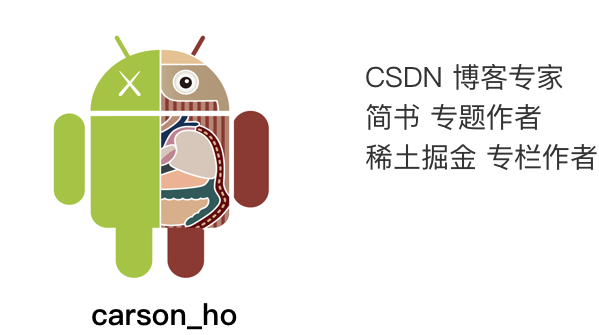# 请帮顶 / 评论点赞！因为你们的赞同/鼓励是我写作的最大动力！©️2019 CSDN 皮肤主题: 编程工作室 设计师: CSDN官方博客